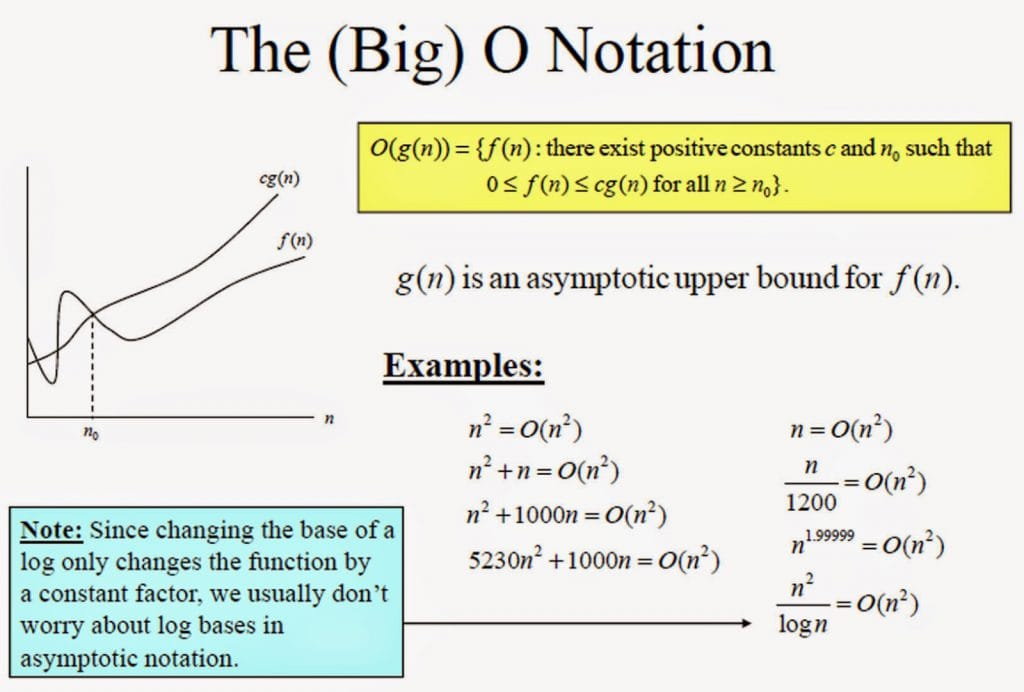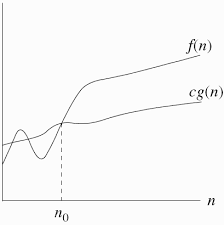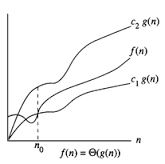# Algorithm Asymptotic Analysis A+ A  A-

in The Previous Post We have Learned What is algorithm? In Computer Science we have some problem which we have to solve, but before writing the actual program, we can write in informal language ,that is called Algorithm, But Before implementing Algorithm in actual sense we have to take care of how much time and memory particular Algorithm will take,Then we will implement the Algorithm in to programming language. So Time and Space Used by Algorithm is the main concern of Design And Analysis of Algorithm. After Analyzing the algorithm we will chose the Best One which will take least Time and Least Memory Requirement for the Program to Execute.

Before Utilizing ans Analysing the Algorithm Lets Get Familiar with Some Notation and Terminology,there are some of the notation used in this, One is Asymtotic Notation ,

First One is Big(oh) Represented By Capital O,

But Before Moving On to Asymptotic Analysis we should come to know  the below things

1.What to Measure

-Time,Space,IO

-Best ,Average,Worst Case?

-We need to Identify What mathematical function we should use?

-What is the Complexity of that Function?

-Defined in terms of Bounds Growth Rate,

This Bounds were expressed using Asymptotic Notations ,We can Make an Analogy Between These Notations and Ordinary Arithmetic Equality and Inequality Relations,So Just a Quick Preview,We are Going to Study Something Called Big O which is Sort of Like O Analogy ≤ (Less then or Equal to)

we can Have Ω Omega which is Analogas to ≥ (Greater then or Equal to)

we can Have Theta Notation, θ which is Analogas to = (equal)

we can Have o(little o) which is analogas to < we can have Ω(Little Omega) which is analogas to >

Now lets Discuss Each one in Some what Details

Big Oh Notation
We express complexity using big-O notation,User to measure running time.Basically, it tells you how fast a function grows or declines.Big O specifically describes the worst-case scenario, and can be used to describe the execution time required or the space used (e.g. in memory or on disk) by an algorithm.For example, when analyzing some algorithm, one might find that the time (or the number of steps) it takes to complete a problem of size n is given by T(n) = 4n^2 – 2 n + 2.

If we ignore constants (which makes sense because those depend on the particular hardware the program is run on) and slower growing terms, we could say “T(n) grows at the order of n^2 ” and write:T(n) = O(n^2).

T(n) = O(f(n)), (pronounced order of or big oh), says that the growth rate of T(n) is less than or equal (<) that of f(n)

Formal Defination
Ο(f(n)) = { g(n) : there exists c > 0 and n0 such that f(n) ≤ c.g(n) for all n > n0. }

Here is a list of classes of functions that are commonly encountered when analyzing algorithms. The slower growing functions are listed first. c is some arbitrary constant.

O(1)          constant

O(log n)   logarithmic

O(n)           linear

O(n log n)      “n log n”

O(n3)          cubic

nO(1)               polynomial

2O(n)             exponential

Omega Notation, Ω>
Ω notation provides an asymptotic lower bound.It is Usefull for finding the Best time an Algorithm can TakeFormal Defination
Ω(f(n)) ≥ { g(n) : there exists c > 0 and n0 such that g(n) ≤ c.f(n) for all n > n0. }

Theta Notation, θ

The notation describes asymptotic tight bounds

A theoretical measure of the execution of an algorithm, usually the time or memory needed, given the problem size n, which is usually the number of items. Informally, saying some equation f(n) = Θ (g(n)) means it is within a constant multiple of g(n). The equation is read, “f of n is theta g of n”.Formal Definition: f(n) = Θ (g(n)) means there are positive constants c1, c2, and k, such that 0 ≤ c1g(n) ≤ f(n) ≤ c2g(n) for all n ≥ k. The values of c1, c2, and k must be fixed for the function f and must not depend on n.

Try Now Data Structure MCQs